## Section49.4Reflection and Refraction

Suppose a monochromatic plane wave in air is incident on a plane surface of glass of refractive index $n$ at an angle $\theta_1\text{.}$ A reflected ray would come back in air at angle $\theta^{\prime}_1$ and a refracted ray would be transmitted into the glass at angle $\theta_2\text{.}$ Can we see this in terms of wave picture of light?

To see the reflection and refraction angles, we only need to look at the wavefronts of the three waves. First, note that frequencies of incident, reflected and refracted light will be equal to each other. But, \since refractive indices of air less than that of glass, wavelength will be larger in air and smaller in glass. Figure 49.4.1 shows these by the spacings between the wavefronts.

### Subsection49.4.1Fresnel's Equations

To study the intensities in the reflected and transmitted waves, we need to actually solve Maxwell's equations for this situation. We represent each wave in the plane wave form. For instance, electric field wave will be

\begin{equation*} \vec E = \vec E_0 \cos(\vec k \cdot \vec r - \omega t), \end{equation*}

where $\vec E_0$ amplitude as vector in the direction of the electric field, $\vec k$ is the wave propagation vector, which is product of wavenumber $k$ and unit vector in the direction of wave, and $\omega$ is the angular frequency of the wave. Here, $\vec r$ is the position and point in space andn $t$ is the instant. The associated magnetic field is similar

\begin{equation*} \vec B = \vec B_0 \cos(\vec k \cdot \vec r - \omega t), \end{equation*}

with the following relation between the amplitudes for wave in vacuum,

\begin{equation*} B_0 = \frac{E_0}{c}, \end{equation*}

and the directions of the electric field, magnetic field, and propagation are related by

\begin{equation*} \vec k\ \ \text{ in the direction of }\ \ \vec E\times \vec B. \end{equation*}

The full calculations using Maxwell's equations to understand relations between the amplitudes and direcions is beyond the scope of this book. I will quote here some of the important results for a monochromatic light.

#### Subsubsection49.4.1.1Plane of Incidence and Polarizations

The amplitudes of reflected and transmitted waves depend on the direction of the electric field. This direction is given with respect to the plane containing the directions of the incident, reflected, and transmitted waves as shown in Figure 49.4.2. This plane is called plane of incidence. If electric field is perpendicular to the plane of incidence, we say the wave is S polarized or transverse electric or TE. If the direction of electric field is in the plane of incidence, then the direction of magnetic field will be perpendicular to the plane of incidence - we call these waves P poarized or transverse magnetic or TM.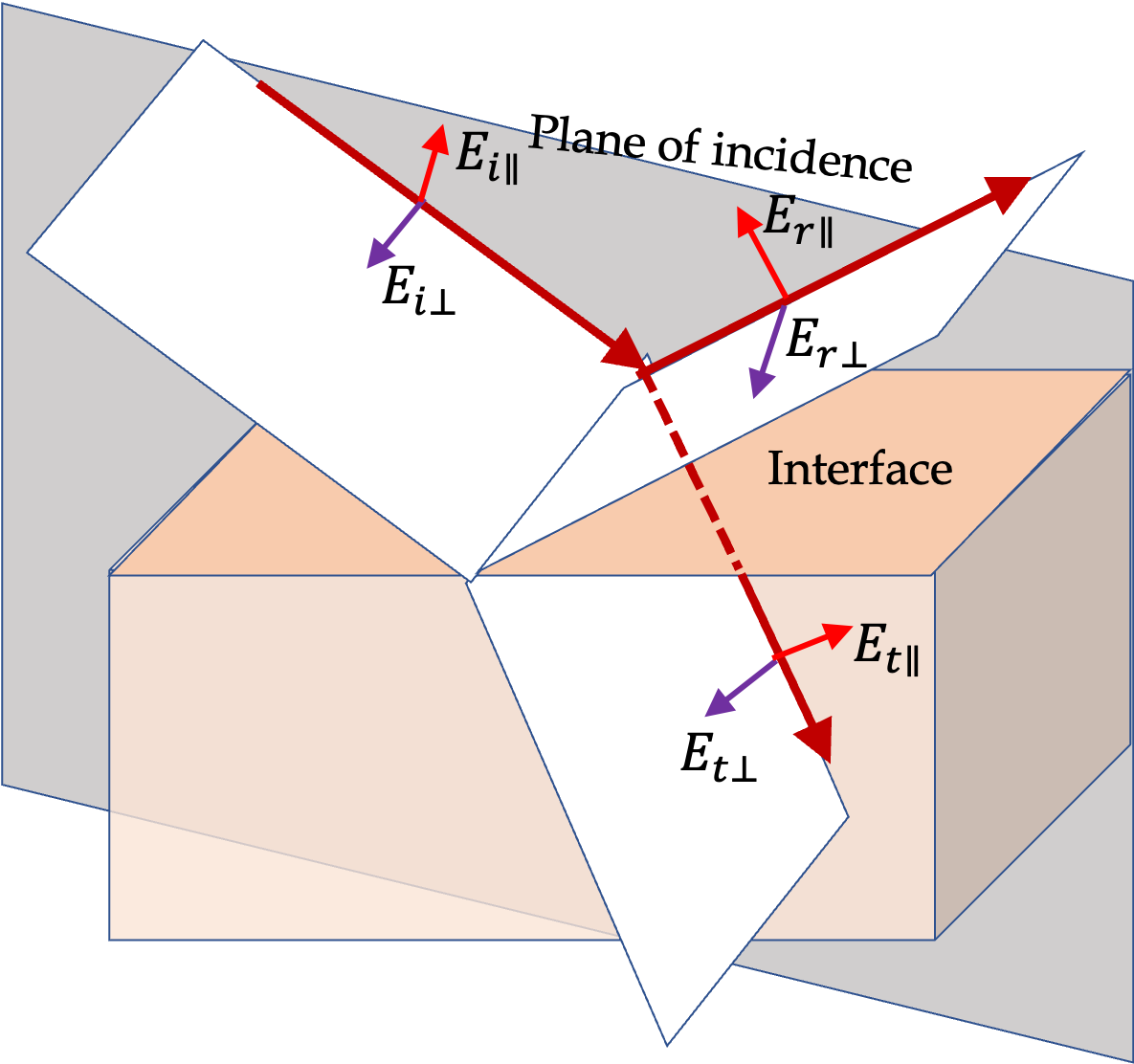Figure 49.4.2. Plane of Incidence and S ($\perp$) and P ($\parallel$) polarizations. S and P polarizations are also called TE and TM polarizations. The electric fields for S polarization are labeled with $\perp\text{,}$ to indicate that they are perpendicular to the plane of incidence and those for P (meaning parallel) polarization are labeled with $\parallel\text{.}$

#### Subsubsection49.4.1.2Reflection and Transmission Coefficients

Let $E_{0i}\text{,}$ $E_{0r}\text{,}$ and $E_{0t}$ be the electric field amplitudes in the incident, reflected and refracted waves respectively. Maxwell's equations require that the component of electric and magnetic fields that are parallel to the interface be same on the two sides of the interface - these are called boundary conditions. Applying these boundary conditions leads to equations that can be solved for reflection amplitudes and transmission amplitudes in terms of incidence amplitudes. Therefore, we define the ratios $E_{0r}/E_{0i}$ and $E_{0t}/E_{0i}$ to write the answers for the two polarization cases. These ratios are called reflection coefficient and transmission coefficient, respectively, and denoted by $\rho$ and $\tau\text{.}$

\begin{align*} \amp \rho = \frac{E_{0r}}{E_{0i}}, \ \ \ \tau = \frac{E_{0t}}{E_{0i}}. \end{align*}

Additionally, boundary conditions also show that the waves must satisfy law of reflection and Snell's law of refraction. With incidence angle $\theta_1\text{,}$ reflected angle $\theta_1^\prime\text{,}$ refracted angle $\theta_2\text{,}$ and the refractive indices $n_1$ and $n_2\text{,}$ by matching of the phases of the three waves we get the usual ray optics laws.

\begin{align*} \amp \theta_1^\prime = \theta_1,\ \ \ n_1\, \sin\theta_1 = n_2\, \sin\theta_2. \end{align*}

#### Subsubsection49.4.1.3Fresnel Equations for S Polarized Waves

Figure 49.4.3 shows rays in the plane of incidence and the direction of electric and magnetic fields in the electromagnetic wave picture of light.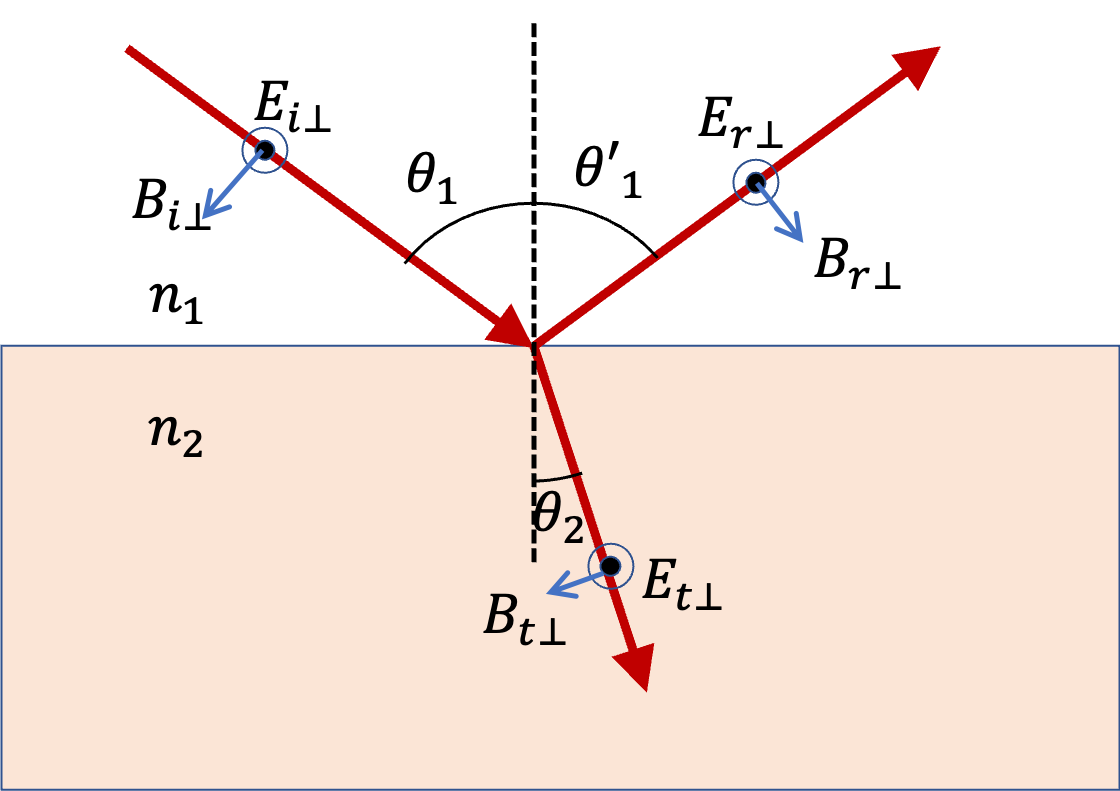Figure 49.4.3. The electric field polarizations for reflection and transmission waves. The boundary condition enforces $E_{i\perp} + E_{r\perp} = E_{t\perp}\text{.}$ When you examine magnetic field, you will find it parallel to the plane. You will need to work out the component of the magnetic field that is parallel to the interface. Suppose horozontal axis is the $x$ axis, then you would enforce $B_{ix\perp}/\mu_1 + B_{rx\perp}/\mu_1 = B_{tx\perp}/\mu_2\text{,}$ where $\mu$ is magnetic susceptibility. Normally we have $\mu_1=\mu_2\approx\mu_0\text{.}$

For S polarized waves we denote the reflection and transmission coefficients as $\rho_\perp (= E_{0r\perp}/E_{0i\perp})$ and $\tau_\perp (= E_{0t\perp}/E_{0i\perp})$ respectively. There are several alternative ways to write these solutions, using Snell's law and the relation between electric susceptibility and refractive index, $\epsilon = n^2\text{.}$ Following are two useful expressions of $\rho_\perp$ and $\tau_\perp$ when we use $\mu_1=\mu_2\approx\mu_0\text{.}$

\begin{align} \rho_{\perp} \amp = \frac{n_1\cos\theta_1 - n_2\cos\theta_2}{n_1\cos\theta_1 + n_2\cos\theta_2} = \frac{\sin(\theta_2-\theta_1)}{\sin(\theta_2+\theta_1)}. \label{eq-rho-for-s-polarization}\tag{49.4.1}\\ \tau_{\perp} \amp = \frac{2n_1\cos\theta_1}{ n_1\cos\theta_1 + n_2\cos\theta_2 } = \frac{2\sin\theta_2 \cos\theta_1}{\sin(\theta_2+\theta_1)}. \label{eq-tau-for-s-polarization}\tag{49.4.2} \end{align}

#### Subsubsection49.4.1.4Fresnel Equations for P Polarized Waves

Figure 49.4.4 shows rays in the plane of incidence and the direction of electric and magnetic fields in the electromagnetic wave picture of light for the case of P-polarization.Figure 49.4.4. The electric field polarizations for reflection and transmission waves. The boundary condition enforces $B_{i\parallel}/\mu_1 + B_{r\parallel}/\mu_1 = B_{t\parallel}/\mu_2\text{.}$ Note that the direction of the reflected magnetic field is flipped compared to the direction of the incident magnetic field \since the direction of the wave is cross-product of electric and magnetic fields. You will need to work out the component of the electric field that is parallel to the interface. Suppose horozontal axis is the $x$ axis, then you would enforce $E_{ix\parallel} + E_{rx\parallel} = E_{tx\parallel}\text{.}$

For P polarized waves we denote the reflection and transmission coefficients as $\rho_\parallel (= E_{0r\parallel}/E_{0i\parallel})$ and $\tau_\parallel (= E_{0t\parallel}/E_{0i\parallel})$ respectively. There are several alternative ways to write $\rho_\parallel$ and $\tau_\parallel\text{,}$ using Snell's law and the relation between electric susceptibility and refractive index, $\epsilon = n^2\text{.}$ Following are two useful expressions of $\rho_\parallel$ and $\tau_\parallel$ when we use $\mu_1=\mu_2\approx\mu_0\text{.}$

\begin{align} \rho_{\parallel} \amp = \frac{n_1\cos\theta_2 - n_2\cos\theta_1}{n_1\cos\theta_2 + n_2\cos\theta_1} = \frac{\tan(\theta_1-\theta_2)}{\tan(\theta_1+\theta_2)}. \label{eq-rho-for-p-polarization}\tag{49.4.3}\\ \tau_{\parallel} \amp = \frac{2n_1\cos\theta_1}{ n_1\cos\theta_2 + n_2\cos\theta_1 } = \frac{2\sin\theta_2 \cos\theta_1)}{\sin(\theta_1+\theta_2)\cos(\theta_1-\theta_2)}.. \label{eq-tau-for-p-polarization}\tag{49.4.4} \end{align}

### Subsection49.4.2Reflection Coefficient, Brewster's and Critical Angles

In Figure 49.4.5 I have plotted reflecion coefficients for reflection when light is incident from air onto glass. Since refractive index of the incident medium is less than that of the second medium, we do not get total internal reflection. Instead, we seen different behaviors of the two polarizations. The S-polarized reflected wave amplitude is negative compared to the incident wave amplitude. With increasing angle, $|\rho_\perp|$ increases to $1$ when incidence angle in $90^\circ\text{,}$ i.e., the ray is just grazing on the glass plate.

The P wave shows some strange behavior. The reflection coefficient actually vanishes at a special angle, called Brewster's angle. At this angle $\rho$ vanishes. Let us denote this special incidence angle by $\theta_B\text{.}$ When we set $\theta_1=\theta_B$ in Eq. (49.4.3) and set that to zero, we find a formula for $theta_B$ in terms of the refractive indices.

\begin{equation*} n_1\sqrt{1- (n_1/n_2)^2 \sin^2\theta_B} - n_2\cos\theta_B = 0. \end{equation*}

You can solve this to find that

\begin{equation*} \sin\theta_B = \frac{n_2}{\sqrt{n_1^2 + n_2^2}}, \end{equation*}

where I ignored negative root \since angle here is positive. We can get $\cos\theta_B$ from it and actually, write a simpler formula for Brewster's angle.

\begin{equation} \tan \theta_B = \frac{n_2}{n_1}.\tag{49.4.5} \end{equation}

For ray from air into glass, we have $n_1=1.0$ and $n_2=1.55\text{.}$ This gives

\begin{equation} \theta_B = \tan^{-1} \left( n_2 / n_1 \right) = \tan^{-1} \left( 1.55 / 1.0 \right) = 57.2^\circ.\tag{49.4.6} \end{equation}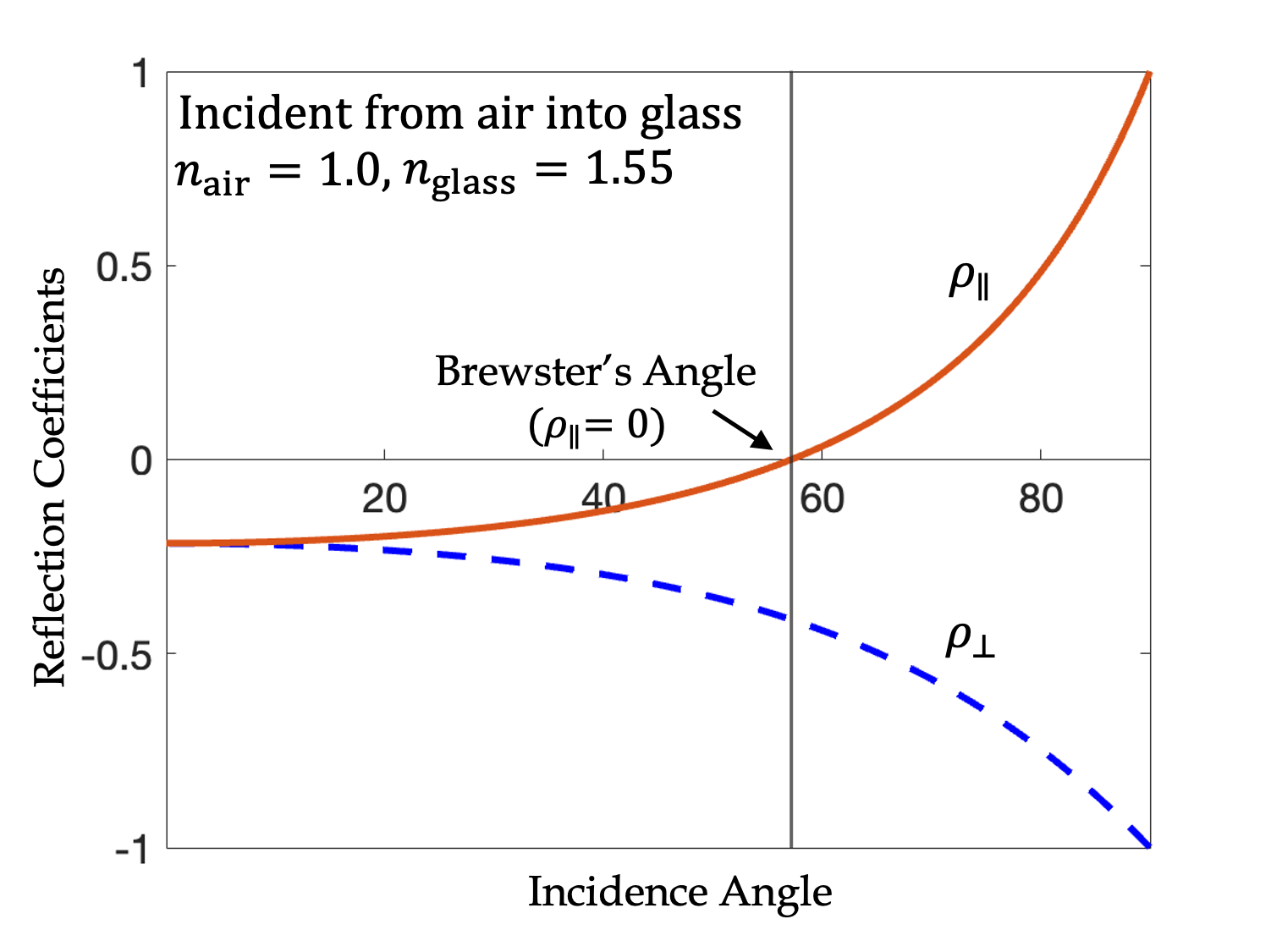Figure 49.4.5. Reflection coefficients for reflection when light is incident from air (lower refractive index medium) on glass (higher refractive index medium) for two types of polarizations. In the S polarization case (subscript $\perp$), the reflected amplitude is negative compared to the incident amplitude; that is, the reflected wave is flipped in phase compared to the incident wave. In the P polarization case (subscript $\parallel$), the reflected amplitude is negative for small values but becomes positive, vanishing at a special angle called Brewster's angle.

To study critical angle, we need to study incidence from a medium of higher refractive index into a medium of lower refractive index. For instance, a ray in glass being incident on glass/air interface. We see that same critical angle $\theta_c$ occurs for both polarizations when the incidence angle is

\begin{equation} \theta_c = \sin^{-1}\left( n_2 / n_1 \right).\tag{49.4.7} \end{equation}

In the present example, we have $n_1=1.55$ and $n_2=1.0\text{.}$ Therefore,

\begin{equation*} \theta_c = \sin^{-1}\left( 1.0 / 1.55 \right) = 40.2^\circ. \end{equation*}

But, only the P-polarization reflected wave vanishes at the Brewster's angle, which will be different the Brewster's angle of $57.5^\circ$ we found above \since the media are switched now.

\begin{equation*} \theta_B = \tan^{-1}\left( n_2 / n_1 \right) = \tan^{-1}\left( 1.0 / 1.55 \right) = 32.8^\circ. \end{equation*}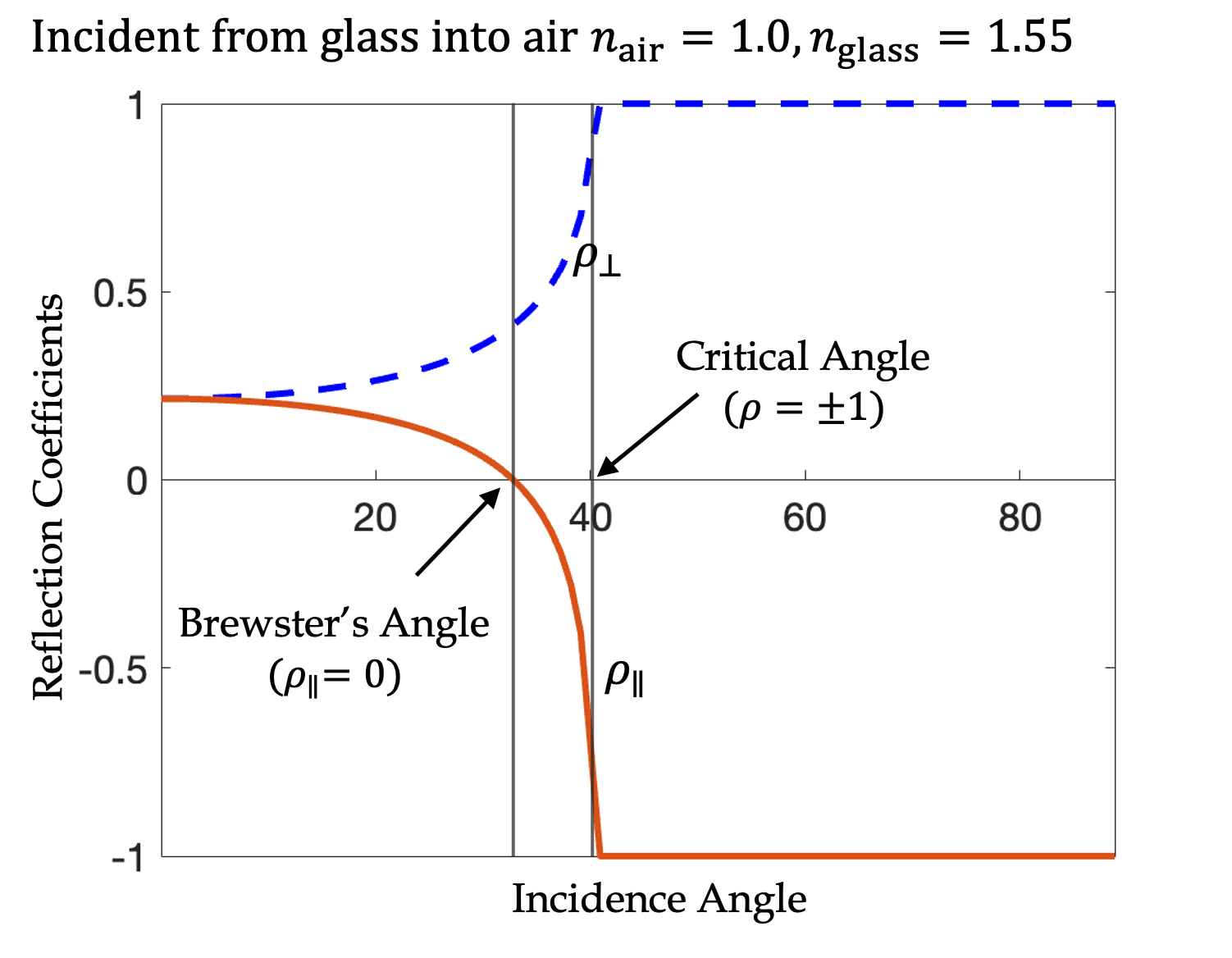Figure 49.4.6. Reflection coefficients for reflection when light is incident from aglass (higher refractive index medium) on air (lower refractive index medium) for two types of polarizations. In the S polarization case (subscript $\perp$), the reflected amplitude is positive and increases with angle till it becomes the max allowed of $+1\text{,}$ i.e., there is no transmitted ray at this point. This incidence angle is called critical angle. It occurs when incidence angle, $\theta_1 = \sin^{-1}\left( n_2/n_1\right)\text{.}$ In the P polarization case (subscript $\parallel$), the reflected amplitude is positive for small values but becomes negative, vanishing at the Brewster's angle; once $\rho_\parallel$ becomes negative it keeps becoming more negative till it reaches the minimum $-1\text{.}$ The incidence angle at which this happens is the same critical angle at which no transmission into air takes place in this case.

### Subsection49.4.3Reflectance and Transmittance

Figure 49.4.7(a) shows incident,reflected, and transmitted beams with area $A$ at the interface and the corresponding cross-sectional areas of the beams $A_i\text{,}$ $A_r\text{,}$ and $A_t\text{.}$ From the geometry of the incident beam shown in figure (b), you can show that

\begin{equation*} A_i = A\cos\theta_1. \end{equation*}

Similarly, for other beams we will get

\begin{equation*} A_r = A\cos\theta_1,\ \ A_t = A\cos\theta_2. \end{equation*}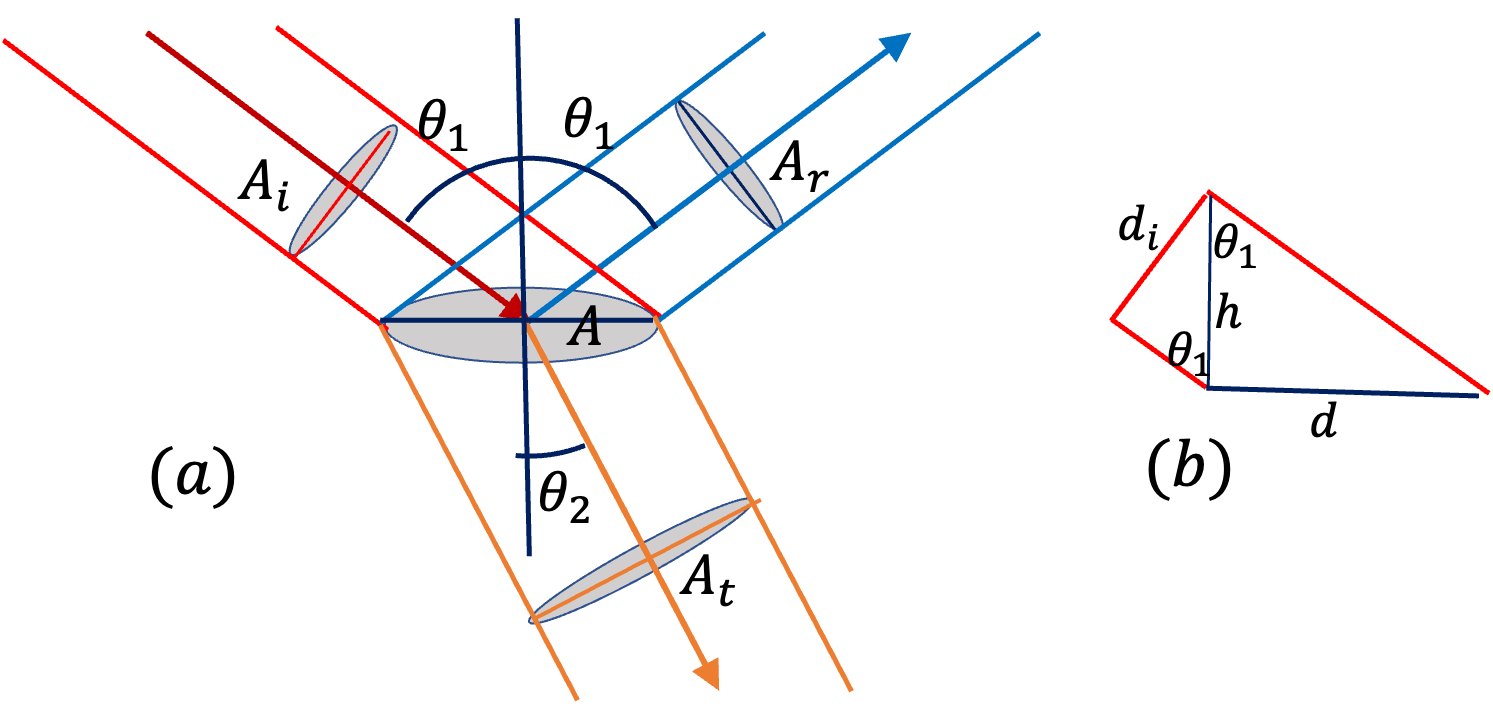Figure 49.4.7. (a) Incident, reflected, and transmitted beams. (b) Geometry for calculating the relation between the area illuminated at the interface and the cross-sectional area of the incident beam.

Now power in each beam will be related to their areas of cross-sections. Let $I_i\text{,}$ $I_r\text{,}$ and $I_t$ be the intensities of the three beams. Recall that intensity in an electromagnetic wave is given by

\begin{equation*} I = \frac{1}{2}\epsilon v E_0^2, \end{equation*}

where $\epsilon$ is the electric susceptibility of the medium, $v$ the speed of light in that medium, and $E_0$ the electric field amplitude of the EM wave. We can write the constant factors multiplying the square of the amplitude by using $\epsilon=n^2$ and $v=c/n$ and get the following useful expression of intensity, which we will use below.

\begin{equation} I = \frac{c}{2} n E_0^2.\tag{49.4.8} \end{equation}

Since intensity is power per unit area, power in each beam will be

\begin{equation*} P_i = I_i A_i,\ \ P_r = I_r A_r,\ \ P_t = I_t A_t. \end{equation*}

From energy conservation, we expect that

\begin{equation} P_r + P_t = P_i\tag{49.4.9} \end{equation}

Dividing both sides by $P_i$ can remove the need to “know” the area of cross-section.

\begin{equation} \frac{P_r}{P_i} + \frac{P_t}{P_i} = 1.\label{eq-energy-conservation-in-terms-of-power-3-beams}\tag{49.4.10} \end{equation}

These ratios are called reflectance and transmittance. They are denoted by $R$ and $T\text{,}$ respectively.

\begin{align} \amp \text{Reflectance, } R = \frac{P_r}{ P_i} = \frac{I_r}{I_i}.\label{eq-reflectance-definition}\tag{49.4.11}\\ \amp \text{Transmitance, } T = \frac{P_t}{ P_i} = \frac{I_t \cos\theta_2}{I_i \cos\theta_1}.\label{eq-transmittance-definition}\tag{49.4.12} \end{align}

Rewriting Eq. (49.4.10), we find that energy conservation in the reflection/refraction of an incident beam then takes a simple form.

\begin{equation} R + T = 1.\tag{49.4.13} \end{equation}

Verifying Energy Conservation

By expressing $R$ and $T$ in terms of intensities and then replacing intensities, we can write $R$ and $T$ in terms of reflection and transmission coefficients, $\rho$ and $\tau\text{.}$

\begin{align*} R \amp = \frac{I_r}{I_i} = \frac{\frac{c}{2} n_1 E_{0r}^2}{\frac{c}{2} n_1 E_{0i}^2} = \rho^2. \\ T \amp = \frac{I_t\cos\theta_2}{I_i\cos\theta_1} = \frac{\frac{c}{2} n_2 E_{0t}^2 \cos\theta_2}{\frac{c}{2} n_1 E_{0i}^2 \cos\theta_1} = \frac{n_2\cos\theta_2}{n_1\cos\theta_1}\ \tau^2. \end{align*}

Let's first verify for S-polarization. Using $\rho$ and $\tau$ from Eqs. (49.4.1) and (49.4.2), we get

\begin{align*} R + T \amp = \rho^2 + \frac{n_2\cos\theta_2}{n_1\cos\theta_1}\ \tau^2 \\ \amp = \left( \frac{n_1\cos\theta_1 - n_2\cos\theta_2}{n_1\cos\theta_1 + n_2\cos\theta_2} \right)^2\\ \amp \ \ \ \ \ \ \ \ \ \ \ \ \ \ \ \ \ \ + \frac{n_2\cos\theta_2}{n_1\cos\theta_1} \left( \frac{2n_1\cos\theta_1}{ n_1\cos\theta_1 + n_2\cos\theta_2 }\right)^2\\ \amp = \frac{(n_1\cos\theta_1 - n_2\cos\theta_2 )^2 + 4n_1n_2\cos\theta_1\cos\theta_2 }{ (n_1\cos\theta_1 + n_2\cos\theta_2 )^2} = 1. \end{align*}

How about for the P-polarization? Using $\rho$ and $\tau$ from Eqs. (49.4.3) and (49.4.4), we get

\begin{align*} R + T \amp = \rho^2 + \frac{n_2\cos\theta_2}{n_1\cos\theta_1}\ \tau^2 \\ \amp = \left( \frac{n_1\cos\theta_2 - n_2\cos\theta_1}{n_1\cos\theta_2 + n_2\cos\theta_1} \right)^2\\ \amp \ \ \ \ \ \ \ \ \ \ \ \ \ \ \ \ \ \ + \frac{n_2\cos\theta_2}{n_1\cos\theta_1} \left( \frac{2n_1\cos\theta_1}{ n_1\cos\theta_2 + n_2\cos\theta_1 }\right)^2\\ \amp = \frac{(n_1\cos\theta_2 - n_2\cos\theta_1 )^2 + 4n_1n_2\cos\theta_1\cos\theta_2 }{ (n_1\cos\theta_2 + n_2\cos\theta_1 )^2} = 1. \end{align*}

### Subsection49.4.4Reflection at Normal Incidence

You saw above that formulas for reflectance $R$ and transmittance $T$ in Eq. (49.4.11) and (49.4.12) become very complicated once we write out the details of the reflection and trtansmission coefficient. They show that $R$ and $T$ depend on incident angle and refractive indices in an unwieldy way. For many applications, such detail is not necessary. A particularly useful case is that of near normal incidence. In that case $\theta_1 \approx 0\text{.}$ Now, with this value of incicenc angle we find that

\begin{align*} \amp R = \left( \frac{n_2 - n_1}{n_2 + n_1} \right)^2,\ \ \ T = \frac{4n_1n_2}{ \left( n_2 + n_1\right)^2 }. \end{align*}

Its much easier to use these formulas to get some rough idea of what will happed at an interface. For instane, at the beach if you looking at the water $(n=1.33)$ from above, you would expect,

\begin{equation*} R = 0.02,\ \ T = 0.98. \end{equation*}

That is, about $2\%$ of light energy will be reflected, the rest will get into the water. Since these equations are symmetric in $n_1$ and $n_2\text{,}$ it does not matter which side of the interface light came from. That is, if you have a light source below the water level, $98\%$ of light energy from that source will also be transmitted in the air.

Suppose refractive index of water and glass are $1.33$ and $1.5$ respectively.

(a) What is the Brewster's angle if incident ray comes from the water side?

(b) What is the Brewster's angle if incident ray comes from the glass side?

Hint

Note that Brewster's angle is given by $\tan^{-1}(n_2/n_1)\text{,}$ where $n_1$ is the incindent side and $n_2$ is the transmitted or refracted side.

(a) $48.4^\circ\text{.}$ (b) $41.6^\circ\text{.}$
(a) Using the formula $\tan^{-1}(n_2/n_1)$ with $n_1$ the refractive index of water and $n_1$ the refractive index of glass we get
(b) Using the formula $\tan^{-1}(n_2/n_1)$ with $n_1$ the refractive index of glass and $n_1$ the refractive index of water we get